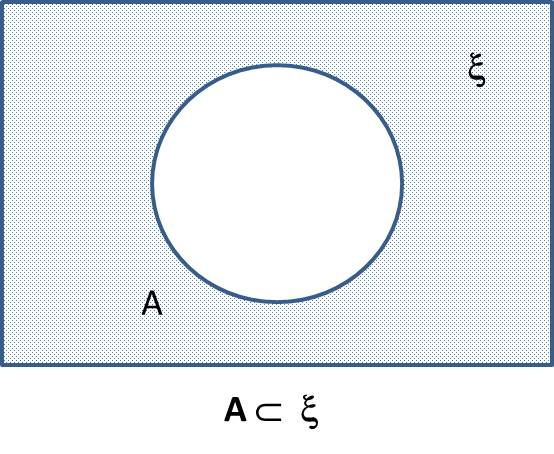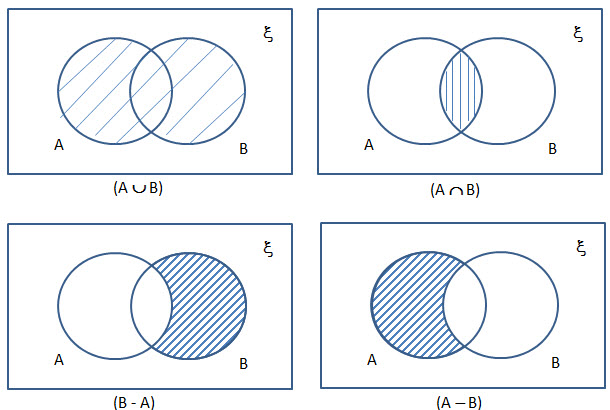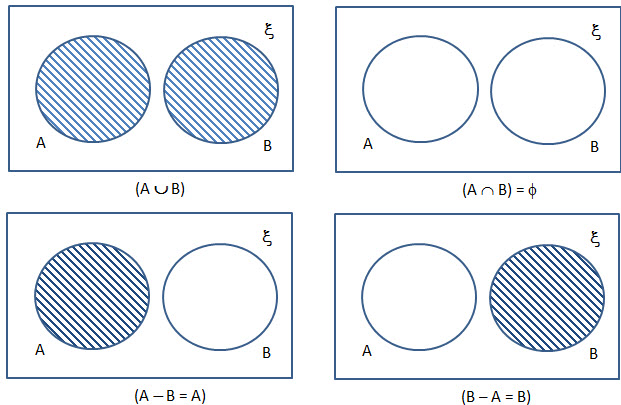Venn Diagrams

Venn diagrams are illustrations to bring out relationships in sets and their use in simple logical problems.

To represent$A \subset \xi$, where$\xi$ is the universal set.To represent two intersecting subset of a universal setTo represent two intersecting subset of a universal setTo represent two disjointed sets of a universal setTo represent two subsets A and B of a universal set such that$A \subset B \xi$To represent three intersecting subset of a universal setImportant result from Venn Diagrams